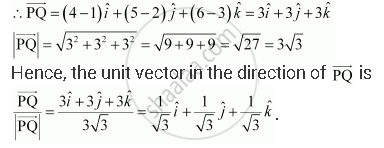Share

# Find the Unit Vector in the Direction of Vector Bar(Pq) , Where P and Q Are the Points (1, 2, 3) and (4, 5, 6), Respectively. - CBSE (Science) Class 12 - Mathematics

#### Question

Find the unit vector in the direction of vector bar(PQ) , where P and Q are the points (1, 2, 3) and (4, 5, 6), respectively.

#### Solution

The given points are P (1, 2, 3) and Q (4, 5, 6).Is there an error in this question or solution?

#### Video TutorialsVIEW ALL 

Solution Find the Unit Vector in the Direction of Vector Bar(Pq) , Where P and Q Are the Points (1, 2, 3) and (4, 5, 6), Respectively. Concept: Properties of Vector Addition.
S